Equations > Chemistry > Atomic Structure

Showing results 1 to 8 of 8, on page 1 of 1
 Description Equation Bohr Radius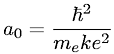Radii of stable orbits in the Bohr model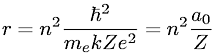Planck's Quantized (Quantum) Energy Equation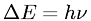Speed of Light to Wavelength and Frequency Relationship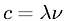De Broglie WavelengthLinear Momentum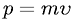Relationship between Energy and Principal Quantum Number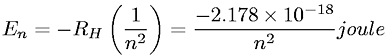Rydberg Equation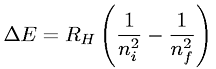(you need to login first)